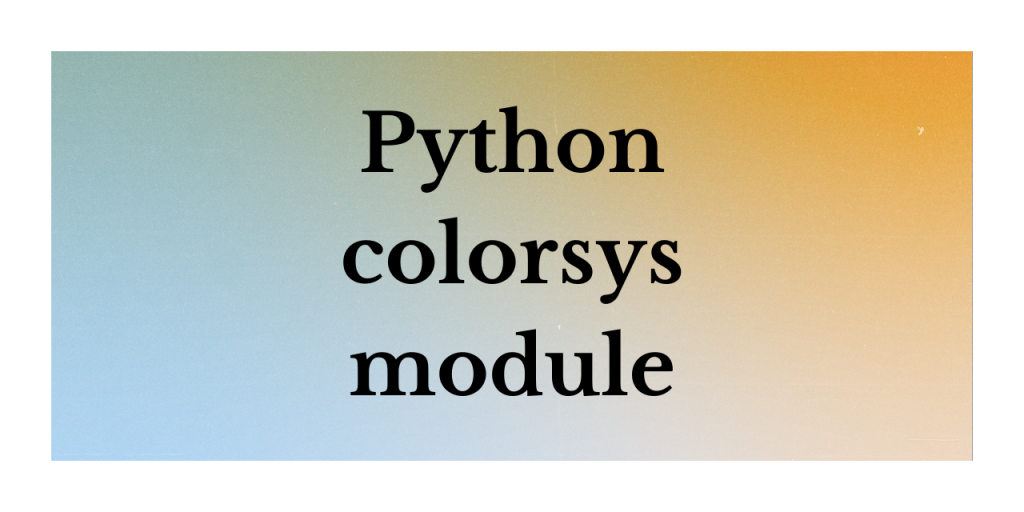# Python colorsys moduleHello, readers! In this article, we will be focusing on Python colorsys module, in detail. So, let us get started! 🙂

## What is the colorsys module?

Python provides us with different modules to test the functionality of the data values and perform manipulations and representations. One such module is Python colorsys module.

The colorsys module help us to perform bi-directional conversion of the below color values–

1. (Hue Lightness Saturation)
2. YIQ (Luminance (Y) In-phase Quadrature)
3. HSV (Hue Saturation Value)
4. RGB (Red, Green, Blue)

The co-ordinate representation of all these colors are floating point values. The permitted range of conversion values is usually between 0 – 1, respectively.

## How to use the colorsys module?

Let us now have a look at their inter-conversions in the upcoming section.

### 1. RGB to YIQ inter-conversion

The colorsys module provides us with rgb_to_yiq() method that initiates the conversion between RGB to Luminance (Y) In-phase Quadrature color ranges. For the same, we need to pass three color values as parameters to the function as shown below:

1. Red
2. Green
3. Blue

Have a look at the below syntax! 🙂

Syntax:

```colorsys.rgb_to_yiq(Red, Green, Blue)
```

Example 1: RGB->YIQ

In the below example, we have passed the three color values Red, Green and Blue to the rgb_to_yiq() function and have resulted into conversion of RGB to YIQ color scale.

```import colorsys

R = 0.1
G = 0.3
B = 0.3

YIQ = colorsys.rgb_to_yiq(R, G, B)

print(YIQ)

```

Output:

```(0.24, -0.11979999999999999, -0.0426)
```

Python colorsys module includes yiq_to_rgb() function that performs the conversion of the Luminance (Y) In-phase Quadrature color values to RGB mode.

Syntax:

```yiq_to_rgb(Y, I, Q)
```

Example 2: YIQ->RGB

We have performed the conversion of YIQ color scale values to Red-Green-Blue color scale.

```import colorsys

Y = 0.1
I = 0.3
Q = 0.3

RGB = colorsys.yiq_to_rgb(Y, I, Q)

print(RGB)

```

Output:

```(0.5711316397228637, 0.0, 0.28013856812933025)
```

### 2. HSV to RGB inter-conversion

Apart from YIQ and RGB, colorsys module offers us with hsv_to_rgb(H,S,V) function to perform conversion of HSV scale data to RGB scale.

Syntax:

```hsv_to_rgb(H,S,V)
```

Example 1: HSV->RGB

```import colorsys

H = 0.1
S = 0.3
V = 0.3

RGB = colorsys.hsv_to_rgb(H, S, V)

print(RGB)

```

Output:

```(0.3, 0.264, 0.21)
```

Apart from this, colorsys module also offers us with rgb_to_hsv(R,G,B) function to perform the conversion of RGB scale to HSV color value format.

Example 2: HSV->RGB

We have made use of rgb_to_hsv() function to perform the conversion of RGB scale to HSV color scale.

```import colorsys

R = 0.1
G = 0.3
B = 0.3

HSV = colorsys.rgb_to_hsv(R, G, B)

print(HSV)

```

Output:

```(0.5, 0.6666666666666666, 0.3)
```

### 3. RGB to HLS inter-conversion

With Python colorsys module, you can easily perform the conversion of RGB color scale to HLS color scale using rgb_to_hls() function.

Syntax:

```rgb_to_hls(R, G, B)
```

Example:

In this example, we have performed the conversion of RGB color scale values to HLS format.

```import colorsys

R = 0.1
G = 0.3
B = 0.3

HLS = colorsys.rgb_to_hls(R, G, B)

print(HLS)

```

Output:

As seen below, and in the above examples as well, the conversion range usually sticks to the scale of 0 to 1 only.

```(0.5, 0.2, 0.49999999999999994)
```

## Conclusion

Feel free to comment below, in case you come across any question. For more such posts related to Python programming, stay tuned with us.

Till then, Happy Learning!! 🙂